## Some Fractals

Fractals are geometric objects that are self-similar, i.e., you can break them apart into smaller pieces which are exact (but smaller) replicas of the original object. These smaller pieces in turn, because they are copies of the original, can be broken up into smaller pieces that are exact (smaller) copies of itself, etc. We see then that a fractal is composed of infinitely many pieces, all of which look the same.

Some fractals are mundane, like a solid square (you can cut it up into 4 smaller squares and each of these squares into 4 smaller squares, etc). But some fractals are extremely complicated, like the ones below.

Since the 'recipe' for producing fractals is so simple (repeat a certain step over an over again on smaller and smaller scales), they are easily drawn by a computer (not exactly of course, because a computer can only draw detail down to some finitely small scale). The two most common ways to draw fractals are using an Iterated Function System (IFS) and the Chaos Game. These are described in the notes on fractals in the link below.

These fractals were drawn with the Fractal Pattern VB program ; the method uses an 'IFS'. Go to the IFS page to see the process of drawing fractals with an IFS. You can also draw these fractals (and more) with the Chaos Game applet. Go to the Chaos Game page to see the process of drawing fractals using the chaos game.

See the W00 course notes for more information about fractals.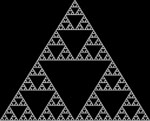The Sierpinski triangle. (Larger view: white on black , black on white.)
One way to draw this is to start with a solid triangle, divide it up into four smaller triangles each one of which has 1/4 the area of the whole triangle, and then remove the middle triangle. Now repeat the procedure with each of the three remaining small triangles. Continue ad infinitum.
The Sierpinski triangle is actually made up just of lines, and if you joined them together they would make a line infinitely long. The area of the Sierpinski triangle is zero (if you added up the areas of all the small triangles you removed during the construction, their areas would add up to the area of the original triangle).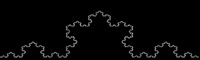The von Koch curve. (Larger view: white on black, black on white.)
One can draw this by starting with __/\__ and then adding a 'bump' to each of the straight sides (i.e., repeat the basic pattern on smaller and smaller scales). Continue ad infinitum. The von Koch curve has infinite length (even though it is contained inside a region of finite area).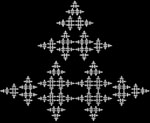The christmas tree fractal. (Larger view: white on black, black on white .) What basic pattern was repeated here to produce this fractal?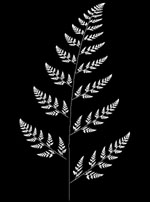The fern. (Larger view: white on black , black on white.)
If you look closely you will see that every 'leaf' of the fern is a tiny fern itself.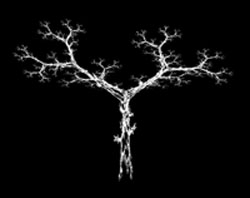A fractal tree. (Larger view: white on black , black on white.) This may not look like a fractal at first sight, but look more closely and you can see the self similarity. To find out how this fractal is drawn, check the 'blue print' of this fractal with the VB program Fractal Pattern , or the applet Fractal Movie.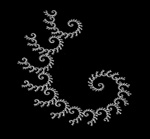A spiral fractal. (Larger view: white on black , black on white.) Even though this fractal (like all the other fractals on this page) was drawn using only linear transformations, the resulting fractal can contain curved lines.